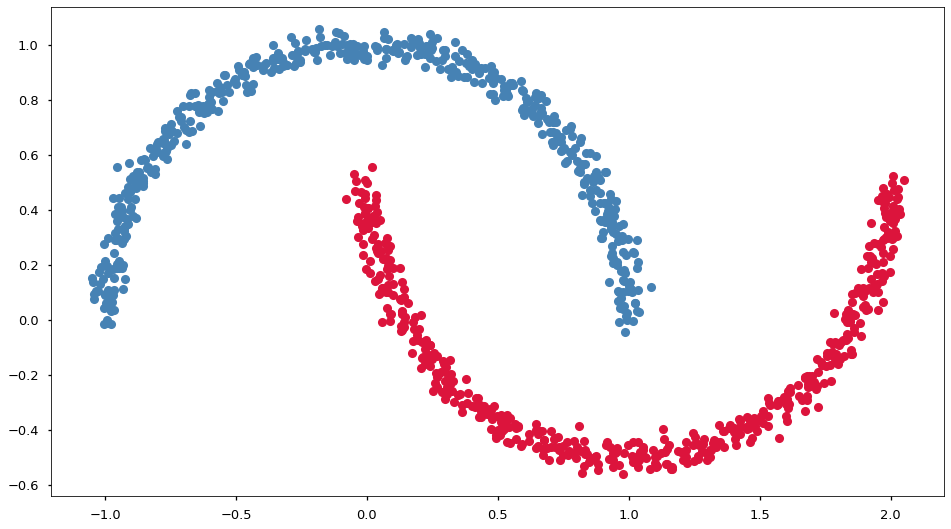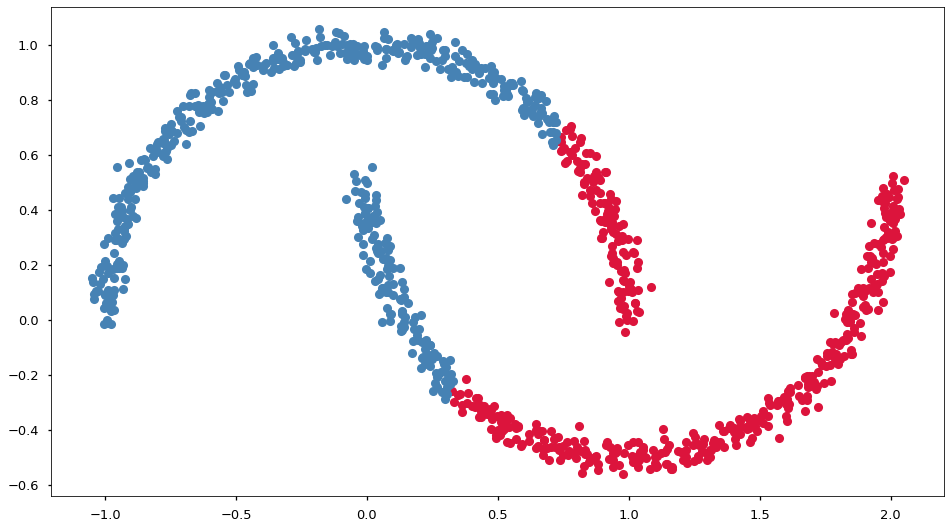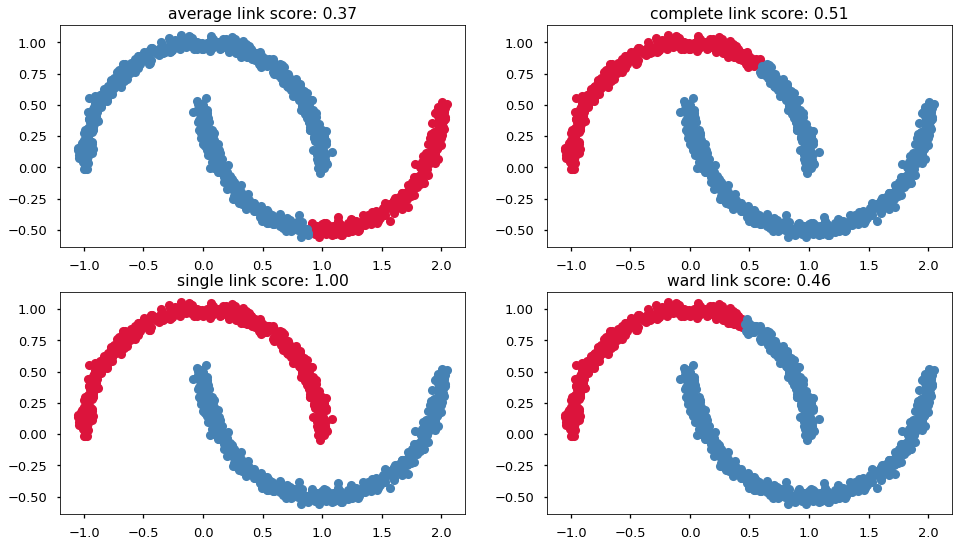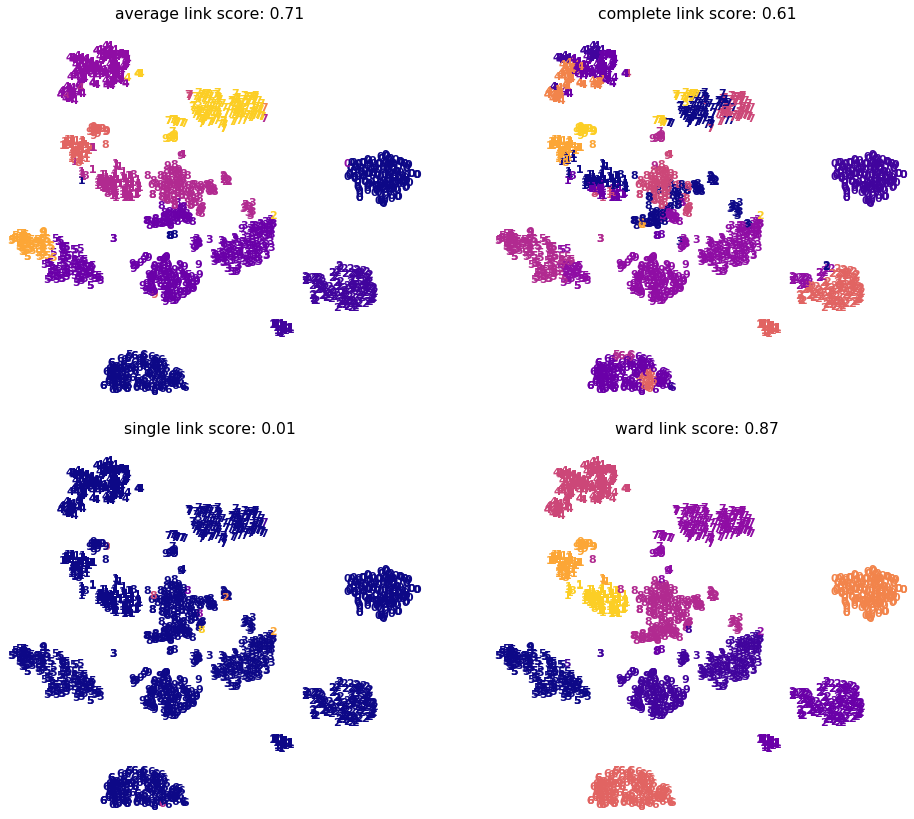# 08.06 Hierarchical¶

There are more clustering techniques out there. Notably techniques that can deal directly with non-linearity to some extent. We will look at a group of clustering techniques that are called Hierarchical or Agglomerative Clustering depending on who is naming it.

We import things we saw before and the Agglomerative Clustering from sklearn. Also, we import make_moons which it a dataset constructor similar to make_blobs we saw before.

In :
import numpy as np
import matplotlib.pyplot as plt
%matplotlib inline
plt.style.use('seaborn-talk')
from sklearn.manifold import TSNE
from sklearn.cluster import AgglomerativeClustering, MiniBatchKMeans
from sklearn.metrics import v_measure_scoreul-moon.svg

The make_moons procedure builds a dataset with a touch of nonlinearity. Here we build two crescent moons with a tiny amount of noise. The dataset construction procedure provides us with labels.

We will use the labels to visually verify what the clustering techniques are doing on the dataset but will not provide the labels to the algorithms. To view the ground truth we will be working with we first plot the entire dataset with the labels originally generated.

In :
X, y = make_moons(1024, noise=.03)
fig, ax = plt.subplots(figsize=(16, 9))
ax.plot(X[y == 1, 0], X[y == 1, 1], 'o', c='crimson')
ax.plot(X[y == 0, 0], X[y == 0, 1], 'o', c='steelblue');For a quick start we will attempt our good friend k-means. We know that the number of clusters is $2$ hence we can easily feed that into k-means.

We again use fit_predict and v_measure to evaluate how well the clustering works. On top of that we plot the classes according to the clusters k-means reached.

In :
model = MiniBatchKMeans(n_clusters=2)
y_hat = model.fit_predict(X)

fig, ax = plt.subplots(figsize=(16, 9))
ax.plot(X[y_hat == 1, 0], X[y_hat == 1, 1], 'o', c='crimson')
ax.plot(X[y_hat == 0, 0], X[y_hat == 0, 1], 'o', c='steelblue')
v_measure_score(y, y_hat)

Out:
0.1830425962550414And k-means is not a good clustering technique for this dataset. This is because k-means depends purely on distance from the cluster center, the clusters k-means produces do not follow the structure of the dataset.

In order to follow the non-linear structure in the two moons we will use a form of clustering that cares about the distances between the points in the dataset rather the distance of every points to an arbitrary point in space.

Agglomerative (Or Hierarchical) clustering works by assigning as many clusters as there are data points in the dataset, and every cluster contains a single sample. Then an iterative algorithms attempts to join the clusters together by following the nest steps:

1. Measure the distance between every pair of clusters
2. Join together the two closest clusters, making them into a single cluster
3. repeat steps 1 and 2 until the desired number of clusters is reached

Note that the clusters have no centers bu only samples, hence joining two clusters together simply means placing the samples in the same cluster. Since there are no centers measuring the distance between clusters becomes a tad complicated. This distance measure is called linkage in hierarchical clustering, and the choice of linkage produces very different clusters. When evaluating the distance between two clusters, each cluster is formed of one or more samples and each sample has a position. All samples in one cluster have a distance to all samples in the second cluster, and linkage is a way to make these distances into a single value.

• single linkage considers the shorter distance as the distance between clusters.

• complete linkage uses the maximum distance.

• average linkage takes the average of all distances.

• ward linkage is a little different, instead of using the distances between the clusters it attempts to minimize the variance of the cluster after the merge. In other words the cluster merge it will perform will be the one which increases the total variances within clusters the least.

The algorithm is called agglomerative because we keep joining the clusters together. And is called hierarchical because we can join all clusters all the way until we remain with only a single cluster. Then the path taken by the algorithm to reach the single cluster can be considered to be a hierarchy of joins. If the number of clusters to find is not too big agglomerative clustering will perform all joins up to a single cluster and then walk the path of the joins to find a place where the desired number of clusters exist. The path of the joins is cached, hence it is possible to ask to cluster into several numbers of clusters repeatedly at minimal cost.

The linkage is given by the appropriately named linkage= argument. In order to compare the performance of the different linkage schemes, we perform each and graph them. We also add the v-measure as the title of each graph for comparison.

In :
fig, ax = plt.subplots(2, 2, figsize=(16, 9))

for axf, link in zip(ax.flat, ['average', 'complete', 'single', 'ward']):
y_hat = model.fit_predict(X)
score = v_measure_score(y, y_hat)
axf.plot(X[y_hat == 1, 0], X[y_hat == 1, 1], 'o', c='crimson')
axf.plot(X[y_hat == 0, 0], X[y_hat == 0, 1], 'o', c='steelblue');When the classes have well defined and also well separated structure single linkage appears to work very well. Other linkages performed rather randomly, some as badly as k-means whilst others somewhere in between. In this dataset the exact score of the other three linkages is heavily dependent on the random noise in the position of the points. But we cannot take at face value that the single linkage work well for every dataset.

A dataset we already did see that we know that the separation between classes is bad is the digits dataset. The issue with that dataset is that it is hard to visualize what is happening in it because the dataset itself is hard to visualize. In order to see what is happening we will perform t-SNE on the digits dataset and will plot the results of our clustering in the t-SNE projection. But we will not give this projection to the agglomerative clustering algorithm, we will give the algorithm the plain data in all $2914$ dimensions.

In :
digits = load_digits()
digits.data.shape, digits.images.shape
tsne = TSNE(n_components=2)
proj = tsne.fit_transform(digits.data)


The v-measure of plain k-means on the digits dataset was slightly above $0.7$. We will again evaluate all four linkage methods on clustering the digits in $2914$ dimensions.

We will plot the results in a similar fashion to the way we displayed the t-SNE projections before. In order to do that we need to scale our data to a unit square so that the plotting engine has an easy time displaying the graph.

In :
def plot_digits(X, y, ax):
x_min, x_max = np.min(X, axis=0), np.max(X, axis=0)
proj_norm = (X - x_min) / (x_max - x_min)

ax.axis('off')
for i in range(len(y)):
ax.text(proj_norm[i, 0], proj_norm[i, 1], str(digits.target[i]),
color=plt.cm.plasma(y[i] / 10),
fontdict=dict(size=11, weight='bold'))

fig, ax = plt.subplots(2, 2, figsize=(16, 14))
for axf, link in zip(ax.flat, ['average', 'complete', 'single', 'ward']):
y_hat = model.fit_predict(digits.data)
score = v_measure_score(digits.target, y_hat)
plot_digits(proj, y_hat, axf)An in the case of digits the single linkage performed exceptionally badly. The classes in the digits dataset are very close to each other therefore single linkage just made $9$ clusters with a single sample each and a $10th$ cluster with all other samples. The ward linkage on the other hand performs very well on this problem.
Do not be mislead by the graphs. The actual data samples are all very close to each other in $2914$ dimensions. The graphs use the t-SNE projection in order for us to be able to visually inspect what the agglomerative clustering graph is doing.